﻿ 离散观测下线性自吸引扩散的最小二乘估计 Least Squares Estimation for Self-Attracting Diffusion with Discrete Observations

Vol. 08  No. 12 ( 2019 ), Article ID: 33431 , 9 pages
10.12677/AAM.2019.812229

Least Squares Estimation for Self-Attracting Diffusion with Discrete Observations

Jingqi Han

School of Mathematics, Physics and Statistics, Shanghai University of Engineering Science, Shanghai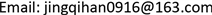Received: Nov. 19th, 2019; accepted: Dec. 6th, 2019; published: Dec. 13th, 2019ABSTRACT

In this paper, the self-attracting diffusion process driven by fractional Brownian motion ${X}_{t}^{H}={B}_{t}^{H}-\theta {\int }_{0}^{t}{\int }_{0}^{t}\left({X}_{s}^{H}-{X}_{u}^{H}\right)duds+vt$ is considered, where ${B}_{t}^{H}$ is fractional Brownian motion with Hurst index $H\in \left[\frac{1}{2},1\right)$, and $\theta >0,v\in ℝ$ are two unknown parameters. With discrete observation, we research the least squares estimators $\stackrel{^}{\theta }$ and $\stackrel{^}{v}$ for the unknown parameters. It is proved that they are not weakly consistency and we also construct some new estimators which have weakly consistency.

Keywords:Fractional Brownian, Least Squares Estimation, Self-Attracting Diffusion1. 引言

${R}_{H}\left(t,s\right)=E\left({B}_{t}^{H}{B}_{s}^{H}\right)\frac{1}{2}\left({s}^{2H}+{t}^{2H}-{|t-s|}^{2H}\right)\text{,}\text{\hspace{0.17em}}\text{\hspace{0.17em}}s,t\ge 0.$

${X}_{t}^{H}={B}_{t}^{H}-\theta {\int }_{0}^{t}{\int }_{0}^{t}\left({X}_{s}^{H}-{X}_{u}^{H}\right)\text{d}u\text{d}s+vt.$

${Y}_{t}^{H}={\int }_{0}^{t}\left({X}_{s}^{H}-{X}_{u}^{H}\right)\text{d}u$，则模型简化为

${X}_{t}^{H}={B}_{t}^{H}-\theta {\int }_{0}^{t}{Y}_{s}^{H}\text{d}s+vt.$ (1)

2. 准备知识

${B}^{H}=\left\{{B}_{t}^{H},t\ge 0\right\}$ 是定义在完备概率空间 $\left(\Omega ,{\mathcal{F}}_{t},Ρ\right)$ 上的Hurst指数为H的标准一维分数布朗运动。本节主要回顾分数布朗运动的一些基本概念和结论 $\mathcal{H}$ 表示与分数布朗运动有关的再生核Hlibert空间，它为示性函数 $\left\{{1}_{\left[0,t\right]},t\in \left[0,T\right]\right\}$ 关于内积

${〈{1}_{\left[0,t\right]},{1}_{\left[0,s\right]}〉}_{\mathcal{H}}=\frac{1}{2}\left({s}^{2H}+{t}^{2H}-{|t-s|}^{2H}\right)$

$\mathcal{H}=\left\{\phi :\left[0,T\right]\to \Re {‖{\mu }_{\phi }‖}_{\mathcal{H}}<\infty \right\},$

${‖\phi ‖}_{\mathcal{H}}^{2}:={\alpha }_{H}{\int }_{0}^{T}{\int }_{0}^{T}\phi \left(t\right)\phi \left(s\right){|t-s|}^{2H-2}\text{d}t\text{d}s$

${‖\phi ‖}_{|\mathcal{H}|}^{2}:={\alpha }_{H}{\int }_{0}^{T}{\int }_{0}^{T}|\phi \left(t\right)||\phi \left(s\right)|{|t-s|}^{2H-2}\text{d}t\text{d}s<\infty ,$

$E\left({\int }_{0}^{T}{\phi }_{s}\text{d}{B}_{s}^{H}{\int }_{0}^{T}{\psi }_{s}\text{d}{B}_{s}^{H}\right)={\alpha }_{H}{\int }_{0}^{T}{\int }_{0}^{T}|\phi \left(t\right)||\psi \left(s\right)|{|t-s|}^{2H-2}\text{d}t\text{d}s$

$f,g:\left[0,T\right]\to ℝ$ 分别是阶为 $\alpha \in \left(0,1\right),\beta \in \left(0,1\right)$$\alpha +\beta >1$ 为Holder连续函数，Young  证明了Riemann-Stieltjes积分(所谓Young积分) ${\int }_{0}^{T}{f}_{s}\text{d}{g}_{s}$ 是存在的。而且，若 $\alpha =\beta \in \left(1/2,1\right)$$F:{ℝ}^{2}\to ℝ$${C}^{1}$ 的，积分 ${\int }_{0}^{.}\frac{\partial F}{\partial f}\left({f}_{u},{g}_{u}\right)\text{d}{f}_{u}$${\int }_{0}^{.}\frac{\partial F}{\partial g}\left({f}_{u},{g}_{u}\right)\text{d}{g}_{u}$ 在Young积分意义下存在，并且有下述公式成立：

$F\left({f}_{t},{g}_{t}\right)=F\left({f}_{0},{g}_{0}\right)+{\int }_{0}^{t}\frac{\partial F}{\partial f}\left({f}_{u},{g}_{u}\right)\text{d}{f}_{u}+{\int }_{0}^{t}\frac{\partial F}{\partial g}\left({f}_{u},{g}_{u}\right)\text{d}g,t\in \left[0,T\right]$ (2)

3. 最小二乘估计量

${X}_{ih}^{H}-{X}_{\left(i-1\right)h}^{H}={B}_{ih}^{H}-{B}_{\left(i-1\right)h}^{H}-\theta h{Y}_{\left(i-1\right)h}^{H}+vh$ (3)

$\begin{array}{l}\underset{i=1}{\overset{n}{\sum }}{|{X}_{ih}^{H}-{X}_{\left(i-1\right)h}^{H}-\left(v-\theta {Y}_{\left(i-1\right)h}^{H}\right)h|}^{2}\\ =\underset{i=1}{\overset{n}{\sum }}\left[{\left({X}_{ih}^{H}-{X}_{\left(i-1\right)h}^{H}\right)}^{2}-2h\left(v-\theta {Y}_{\left(i-1\right)h}^{H}\right)\left({X}_{ih}^{H}-{X}_{\left(i-1\right)h}^{H}\right)+{h}^{2}\left({v}^{2}-2v\theta {Y}_{\left(i-1\right)h}^{H}+{\theta }^{2}{\left({Y}_{\left(i-1\right)h}^{H}\right)}^{2}\right)\right]\end{array}$ (4)

$\begin{array}{l}{\stackrel{⌢}{\theta }}_{nh}=\frac{\underset{i=1}{\overset{n}{\sum }}\left({X}_{ih}^{H}-{X}_{\left(i-1\right)h}^{H}\right)\underset{i=1}{\overset{n}{\sum }}{Y}_{\left(i-1\right)h}^{H}-n\underset{i=1}{\overset{n}{\sum }}\left({X}_{ih}^{H}-{X}_{\left(i-1\right)h}^{H}\right){Y}_{\left(i-1\right)h}^{H}}{nh\underset{i=1}{\overset{n}{\sum }}{\left({Y}_{\left(i-1\right)h}^{H}\right)}^{2}-h{\left(\underset{i=1}{\overset{n}{\sum }}{Y}_{\left(i-1\right)h}^{H}\right)}^{2}},\\ {\stackrel{⌢}{v}}_{nh}=\frac{1}{nh}\underset{i=1}{\overset{n}{\sum }}\left({X}_{ih}^{H}-{X}_{\left(i-1\right)h}^{H}\right)+\frac{{\stackrel{⌢}{\theta }}_{nh}}{n}\underset{i=1}{\overset{n}{\sum }}{Y}_{\left(i-1\right)h}^{H}\end{array}$ (5)

$\begin{array}{c}{\stackrel{⌢}{\theta }}_{nh}-\theta =\frac{\underset{i=1}{\overset{n}{\sum }}\left({X}_{ih}^{H}-{X}_{\left(i-1\right)h}^{H}\right)\underset{i=1}{\overset{n}{\sum }}{Y}_{\left(i-1\right)h}^{H}-n\underset{i=1}{\overset{n}{\sum }}\left({X}_{ih}^{H}-{X}_{\left(i-1\right)h}^{H}\right){Y}_{\left(i-1\right)h}^{H}}{nh\underset{i=1}{\overset{n}{\sum }}{\left({Y}_{\left(i-1\right)h}^{H}\right)}^{2}-h{\left(\underset{i=1}{\overset{n}{\sum }}{Y}_{\left(i-1\right)h}^{H}\right)}^{2}}-\theta \\ =\frac{\underset{i=1}{\overset{n}{\sum }}\left({B}_{ih}^{H}-{B}_{\left(i-1\right)h}^{H}\right)\underset{i=1}{\overset{n}{\sum }}{Y}_{\left(i-1\right)h}^{H}-n\underset{i=1}{\overset{n}{\sum }}\left({B}_{ih}^{H}-{B}_{\left(i-1\right)h}^{H}\right){Y}_{\left(i-1\right)h}^{H}}{nh\underset{i=1}{\overset{n}{\sum }}{\left({Y}_{\left(i-1\right)h}^{H}\right)}^{2}-h{\left(\underset{i=1}{\overset{n}{\sum }}{Y}_{\left(i-1\right)h}^{H}\right)}^{2}}\\ =\frac{{B}_{nh}^{H}\underset{i=1}{\overset{n}{\sum }}{Y}_{\left(i-1\right)h}^{H}-n\underset{i=1}{\overset{n}{\sum }}\left({B}_{ih}^{H}-{B}_{\left(i-1\right)h}^{H}\right){Y}_{\left(i-1\right)h}^{H}}{nh\underset{i=1}{\overset{n}{\sum }}{\left({Y}_{\left(i-1\right)h}^{H}\right)}^{2}-h{\left(\underset{i=1}{\overset{n}{\sum }}{Y}_{\left(i-1\right)h}^{H}\right)}^{2}}\\ =\frac{{B}_{nh}^{H}\underset{i=1}{\overset{n}{\sum }}{Y}_{\left(i-1\right)h}^{H}-n\underset{i=1}{\overset{n}{\sum }}\left({B}_{ih}^{H}-{B}_{\left(i-1\right)h}^{H}\right){Y}_{\left(i-1\right)h}^{H}}{\Phi \left(n,H\right)},\end{array}$ (6)

${\stackrel{⌢}{v}}_{nh}-v=\frac{1}{nh}\underset{i=1}{\overset{n}{\sum }}\left({B}_{ih}^{H}-{B}_{\left(i-1\right)h}^{H}\right)+\frac{{\stackrel{⌢}{\theta }}_{nh}-\theta }{n}\underset{i=1}{\overset{n}{\sum }}{Y}_{\left(i-1\right)h}^{H}=\frac{{B}_{nh}^{H}}{nh}+\left({\stackrel{⌢}{\theta }}_{nh}-\theta \right)\frac{\underset{i=1}{\overset{n}{\sum }}{Y}_{\left(i-1\right)h}^{H}}{n}.$ (7)

${Y}_{s}^{H}={\int }_{0}^{s}\left({X}_{s}^{H}-{X}_{u}^{H}\right)\text{d}u=s{X}_{s}^{H}-{\int }_{0}^{s}{X}_{u}^{H}\text{d}u={\int }_{0}^{s}u\text{d}{X}_{u}^{H}$ (8)

$\text{d}{X}_{u}^{H}=\left(v-\theta {Y}_{u}^{H}\right)\text{d}u+\text{d}{B}_{u}^{H}$ (9)

$\text{d}{Y}_{S}^{H}=-\theta s{Y}_{S}^{H}\text{d}s+vs\text{d}s+s\text{d}{B}_{S}^{H},$

$\frac{1}{n}\underset{i=1}{\overset{n}{\sum }}{Y}_{ih}^{H}\stackrel{a.s.}{\to }\frac{v}{\theta }\text{.}$ (10)

$n\to \infty ,h\to 0$ 时，有 $h\underset{i=1}{\overset{n}{\sum }}{Y}_{ih}^{H}~{\int }_{0}^{nh}{Y}_{S}^{H}\text{d}s$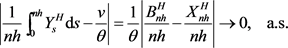(11)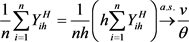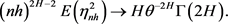(12)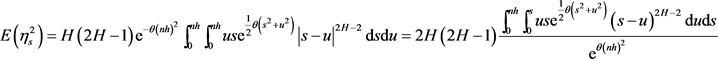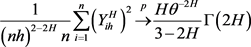(13)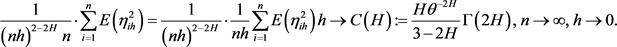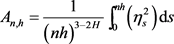，容易得到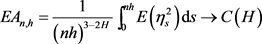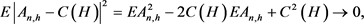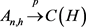。进一步，有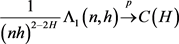(14)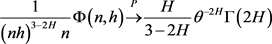(15)(16)。引理得证。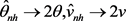Least Squares Estimation for Self-Attracting Diffusion with Discrete Observations[J]. 应用数学进展, 2019, 08(12): 1993-2001. https://doi.org/10.12677/AAM.2019.812229

1. 1. Mishura, Y.S. (2008) Stochastic Calculus for fractional Brownian Motion and Related Processes. Lecture Notes in Mathematics, 1929, Springer, Berlin.
https://doi.org/10.1007/978-3-540-75873-0

2. 2. Nualart, D. (2006) Malliavin Calculus and Related Topics. 2nd Edition. Springer-Verlag, Berlin.

3. 3. Durrett, R. and Rogers, L.C.G. (1992) Asymptotic Behavior of Brownian Polymer. Probability Theory and Related Fields, 92, 337-349.
https://doi.org/10.1007/BF01300560

4. 4. Cranston, M. and Le Jan, Y. (1995) Self-Attracting Diffusions: Two Case Studies. Mathematische Annalen, 303, 87-93.
https://doi.org/10.1007/BF01460980

5. 5. Yan, L., Sun, Y. and Lu, Y. (2008) On the Linear Fractional Self-Attracting Diffusion. Journal of Theoretical Probability, 21, 502-516.
https://doi.org/10.1007/s10959-007-0113-y

6. 6. Hu, Y. and Nualart, D. (2010) Parameter Estimation for Fractional Ornstein-Uhlenbeck Processes. Statistics & Probability Letters, 80, 1030-1038.
https://doi.org/10.1016/j.spl.2010.02.018

7. 7. Gan, Y. and Yan, L. (2018) Least Squares Estimation for a Linear Self-Repelling Diffusion Driven by Fractional Brownian Motion. Science China Mathematics, 48, 1143-1158.
https://doi.org/10.1360/SCM-2017-0387

8. 8. Young, L.C. (1936) An Inequality of Holder Type Connected with Stieltjes Integration. Acta Mathematica, 67, 251-282.
https://doi.org/10.1007/BF02401743